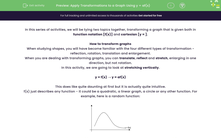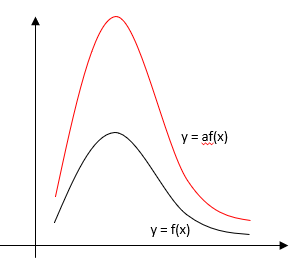# Apply Transformations to a Graph Using y = af(x)

In this worksheet, students will practise applying the transformation y = af(x) to a function defined as y = f(x)Key stage:  KS 4

Year:  GCSE

GCSE Subjects:   Maths

GCSE Boards:   AQA, Eduqas, OCR, Pearson Edexcel,

Curriculum topic:   Algebra, Graphs of Equations and Functions

Curriculum subtopic:   Graphs Transformation of Curves and Their Equations

Difficulty level:#### Worksheet Overview

In this series of activities, we will be tying two topics together, transforming a graph that is given both in function notation [f(x)] and cartesian [y = ].

How to transform graphs

When studying shapes, you will have become familiar with the four different types of transformation - reflection, rotation, translation and enlargement.

When you are dealing with transforming graphs, you can translate, reflect and stretch, enlarging in one direction, but not rotation.

In this activity, we are going to look at stretching vertically.

y = f(x) → y = af(x)

This does like quite daunting at first but it is actually quite intuitive.

f(x) just describes any function - it could be a quadratic, a linear graph, a circle or any other function. For example, here is a random function:If we apply the transformation y = af(x), we are multiplying every value of the function by a (which could be any value) after we have evaluated the function. This means that the value of y will be a times larger than in the original function. This will make the graph stretch in a vertical direction with a scale factor of a.The function y = af(x) is a stretch in a vertical direction with a scale factor of a.

How to apply this to a graph

Example:

You are given the function y = x2 - 4

Sketch the graph of y = 2(x2 – 4)

In the transformation y = af(x), a = 2 so every value of y will double and the values of x will stay the same.Sometimes when you translate a function, you will be expected to label the significant points that have been translated.

This only needs to be done if it is specifically asked for.

Let's try some questions now.

### What is EdPlace?

We're your National Curriculum aligned online education content provider helping each child succeed in English, maths and science from year 1 to GCSE. With an EdPlace account you’ll be able to track and measure progress, helping each child achieve their best. We build confidence and attainment by personalising each child’s learning at a level that suits them.

Get started